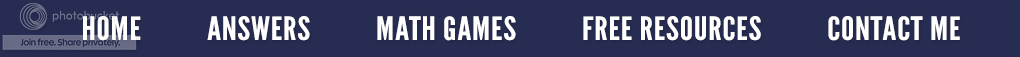### Quick Times - Multiplication Tricks

I am always looking for different strategies when working with my remedial college students since many of the ways they were taught to do math aren't working for them.  I came across this "Quick Times" method and thought it would be another approach I could share with my mathphobics for multiplying.  They love anything that is different, quick and makes them look astute when doing mathematics.

Let's assume we have the multiplication problem of 41 x 12.  In the Quick Times method, first start by multiplying the first digit of 41 by the first digit of 12 to get the first digit of our answer.  We then multiply the second digit of 41 by the second digit of 12 as seen below to get the last digit of our answer (the ones place).

Now we need to find the middle digit of the product.  This is done by multiplying the outside digits, then the inside digits, and adding those two products together as shown below.

This quick method will only work when multiplying two digit numbers by two digit numbers, but it does cause the students to do mental math.  My students like the challenge of doing all of the computation in their heads.  Let's try another one that is a little different.  Let's do 63 x 41.  Again we multiply the first digit of each number and then the second digit of each number to get the first digits of the answer and the last digit of the answer.

As before, multiply the outside digits, then the inside digits, and add the two products together.
Now we must put the 18 into the middle spot, but there is only room for one digit in the tens place.  YIKES!!  What do we do now?  Very easy....because we can only have one digit where the question mark is, we must regroup (carry) the one in the tens place of the 18 and then add it to the 24.

Have you figured out the final answer?  It is.....

You are probably thinking the old method works so much better, but that is only because that is the method you are use to using.  Why not try the ones below using the Quick Times method and see if you get the correct answer.  Use the old method or a calculator to check your answers or go the the answer page above.

a)  36 x 21       b)  24 x  12      c)  48 x 29       d)  59 x 18       e)  63 x 13